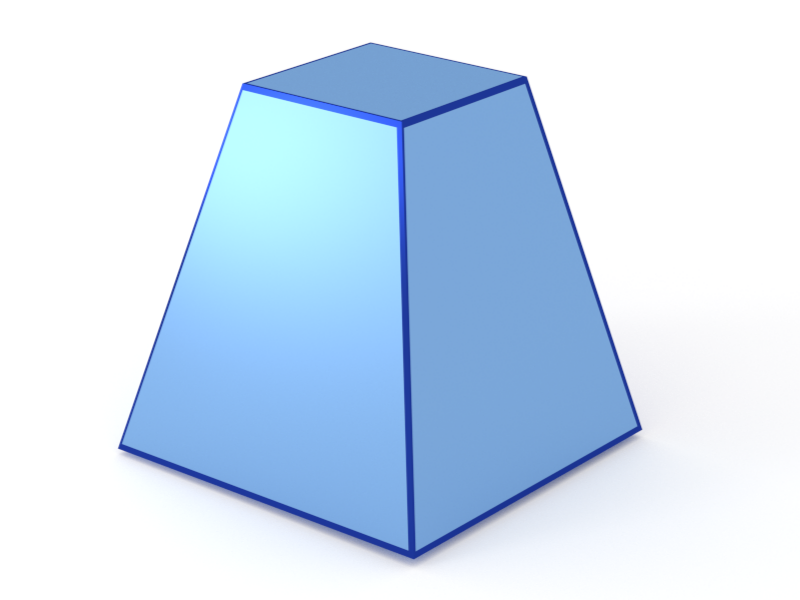### Pyramid4

A truncated pyramid (frustum) with quadratic base.#### Constructor

Pyramid4(L, H, alpha)


Parameters:

• L, edge length of the quadratic base
• H, height
• alpha, dihedral angle $\alpha$ between the base and a side face.

Constraint:

$H \le \dfrac{\tan\alpha}{2} L$

#### Usage

As for any other Form factor.

#### Implementation

Class Pyramid4 inherits from the interface class IFormFactor .

Form factor computation is based on the generic form factor of a polyhedron provided by libformfactor .

Volume has been validated against $$V=\dfrac{L^3\tan\alpha}{6}\Big[1-\Big(1-\dfrac{2H}{L\tan\alpha}\Big)^3\Big].$$

More general:

#### Example

Scattering by uncorrelated, oriented pyramids for horizontal incidence. Rotation around $z$ axis:Generated by Examples/ff/Pyramid4.py .

#### History

Was named “Pyramid” up to BornAgain 1.19.

Corresponds to “Pyramid” form factor of IsGISAXS [manual, Eq. 2.25] and “Square basis pyramid” [Renaud 2009, Eq. 215], except for different parametrization $L = 2R_{IsGISXAXS}$ and a corrected sign.

Reimplemented in BornAgain 1.6 using the generic form factor of a polyhedron [Wuttke 2021], to achieve numerical stability near the removable singularity at $q \to 0$.